Instructor: Maria Blojay

Maria has taught College Algebra and has a master's degree in Education Administration.

This lesson will show how to solve radical inequalities using radical definitions and properties. Key terms and radical notes are included to share step by step the method to solve these special types of inequalities. Updated: 12/10/2021

There are some mathematical definitions and properties for radicals that we need to review. These properties will help us to understand how to solve radical inequalities.

The definition of a radical inequality is an inequality that holds a variable expression within it. This means that the variable expression sits underneath the radical, and is called a radicand.

For example: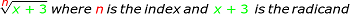Let's take a look at this property: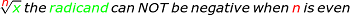The radicand cannot have a calculated final value that is negative WHEN the index of the radical is an even number.

Having a negative under the radical when the index is an even number, such as 2, 4, 6, etc. means that there is no solution. This must be checked for each radical inequality problem. We will see some problems using these extra checks.

We cannot mathematically solve the problem with the radical symbol in it. It must be removed. So don't forget that we have this property that tells us how to do that: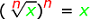This means that we take the index value and use this same value as an exponent. In doing so, it cancels out the radical symbol and leaves the radicand variable expression by itself.

Let's take a look at an example of this: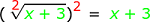If the index is not shown in the radical, it means that the index is equal to two.

Now that we have looked at our radical definitions and properties, we will take a look at three sample problems.

## Example 1

Let's take a look at our first problem: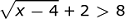In simplifying: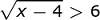Squaring each side, we get,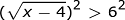Leaving us with,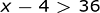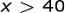To check, choose an x-value greater than 40. If we chose a number such as 68 and replaced this selected value for x: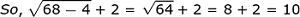which is greater than 8. So x >40 is the correct inequality answer.

## Example 2

Given our second example: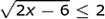To get rid of the radical, we square each side of the inequality: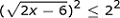We then simplify the inequality and get: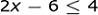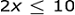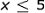Remember that our radicand can NOT be negative, or another way of saying this is that the radicand must be positive:

To check this ... we get: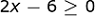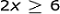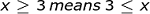Let's check our example with x-values of 3 and 5: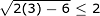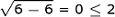Here we have shown this is a true inequality, 0 is less than 2. Now let's try the x value 5: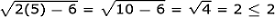Yes, we have a true inequality with an x value of 3 which is equal to 2.

The values of x that are 3 and 5 AND all values of x in between 3 and 5 will make the inequality true.

Here, 3 is our lower interval value, and 5 is our upper interval value.

To write all of the values that would solve this inequality concisely, we would write it as a compound inequality.

A compound inequality is written by using the lower and upper interval values with the same inequality symbols in between those values.

So, we would write: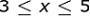## Example 3

In our last example, we have a radical expression on each side of the inequality.

Given: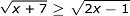Squaring each side:Leaving us with: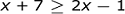To unlock this lesson you must be a Study.com Member.

### Register to view this lesson

Are you a student or a teacher?